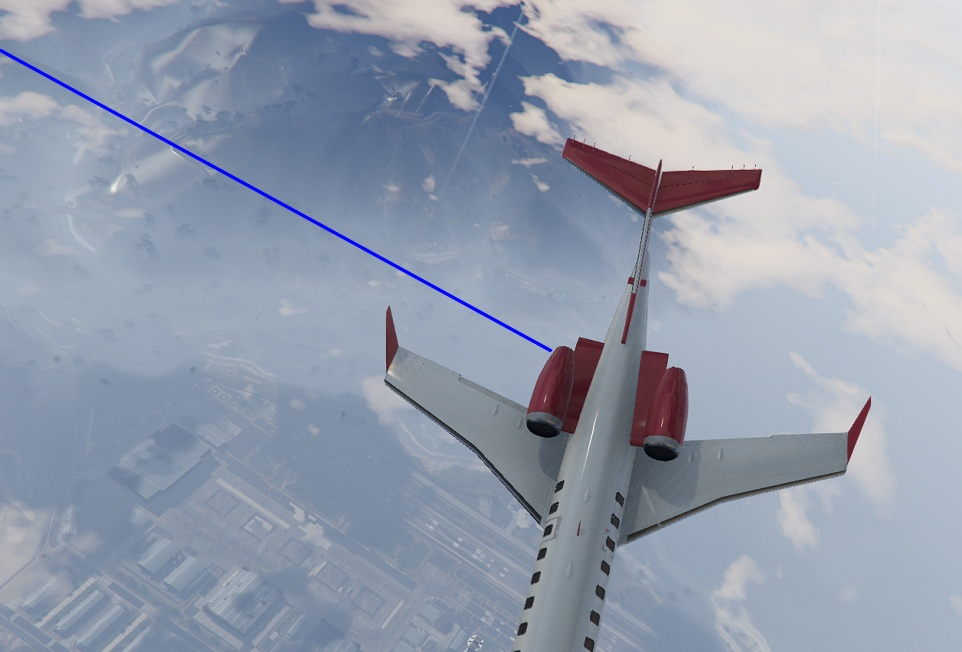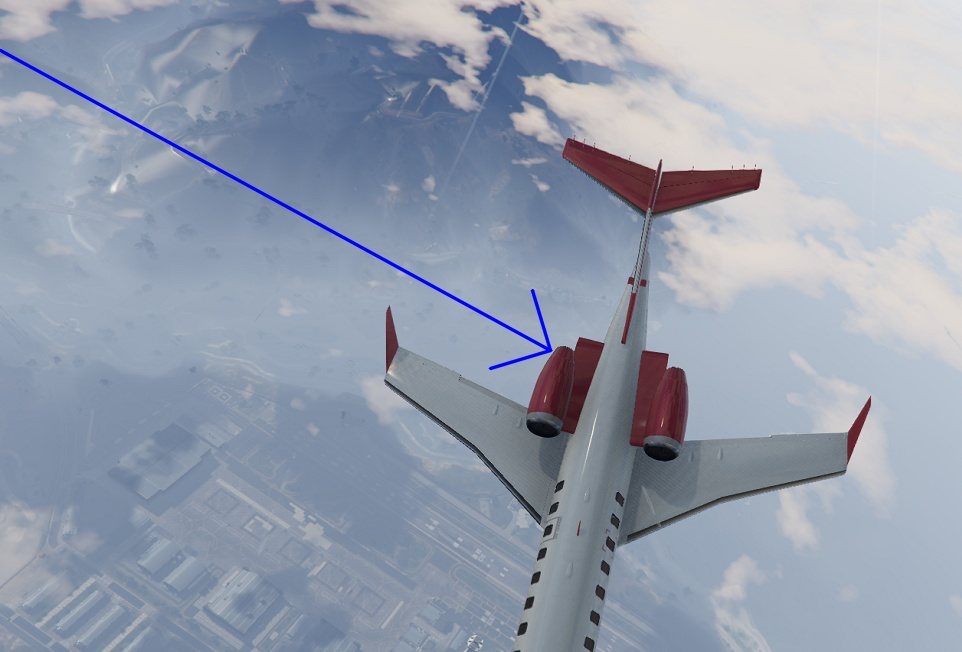Opencv is widely used for processing images. We can use opencv opencv to draw different lines like arrowedLine, Straight Line or Polylines. We will demonstrate line and arrowedLine in this example and for ployLines you can view in this post.

### Draw line

cv2.line() method is used to drawing line on images. We have to provide some arguments to it to draw a line with certain properties on image. These are the arguments that we can pass to method while drawing line.

• Image
• Point 1 (xmin, ymin) - Starting point of line on image
• Point 2 (xmax, ymax) - Ending Point of line on image
• Line Color (B, G, R) - Image color in BGR format
• Line Thickness - Thickness of line as number
• Line Type - Line type, could be `FILLED`, `LINE_4`, `LINE_8`, `LINE_AA`. View More

So, we read an image and draw line on that image using opencv python.

``````# Import cv2 and read image file
import cv2

# Line starting point
point1 = (0, 50)

# Ending Point
point2 = (600, 400)

# Line Color in BGR Format
color = (255, 0, 0) # will be Blue

# Thickness and line type
thickness = 2
linetype = cv2.LINE_AA

# Draw line on image
image = cv2.line(image, point1, point2, color, thickness, linetype)

# show image
cv2.imshow("image", image)
cv2.waitKey(0)
cv2.destroyAllWindows()``````

This will draw a line on image from `(0, 50)` to `(600, 400)` pixels.### Draw Arrowed Line

CV2 also provides another option of arrowed line wich show an arrowed tip at end of line. We can use all configurations as discussed in above simple line. For arrowed line, we just need to call `cv2.arrowedLine()` method instread of `cv2.line()`. There is one other argument named tipLength in arrowedLine method which is not required but we can modify it.

• tipLength - It is the lenght of tip in relation to lenght of arrow length

Now we can create a arrowed line using opencv method on existing image using previous configurations.

``````# arrowed line method
image = cv2.arrowedLine(image, point1, point2, color, thickness, linetype)``````